# Tag: pandas

## pandas filter

Filtering rows of a DataFrame is an almost mandatory task for Data Analysis with Python. Given a Data Frame, we may not be interested in the entire dataset but only in specific rows.

Related course:
Data Analysis with Python Pandas

Filter using query
A data frames columns can be queried with a boolean expression. Every frame has the module query() as one of its objects members.

We start by importing pandas, numpy and creating a dataframe:

This will create the data frame containing: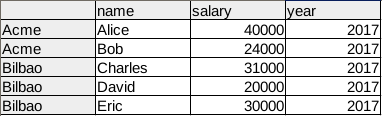After creation of the Data Frame, we call the query method with a boolean expression. This expression is based on the column names that we defined as ‘ABCD’. The query method will return a new filtered data frame.

Total code of data frame creation and filter using boolean expression:

Filter by indexing, chain methods
Instead of queries, we can use in-dices.
We do that by using an array index with boolean expressions:

This will return: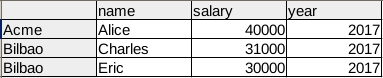Related course:
Data Analysis with Python Pandas

## pandas groupby

DataFrames data can be summarized using the groupby() method. In this article we’ll give you an example of how to use the groupby method.

This tutorial assumes you have some basic experience with Python pandas, including data frames, series and so on. If you are new to Pandas, I recommend taking the course below.

Related course:
Data Analysis with Python and Pandas: Go from zero to hero

## What does groupby do?

The idea of groupby() is pretty simple: create groups of categories and apply a function to them. Groupby has a process of splitting, applying and combining data.

• splitting: the data is split into groups
• applying: a function is applied to each group
• combining: the results (data of different datasets is combined after applying groupby, resulting in a new data structure)

If you programmed databases (SQL) before, you may be familiar with a query like this:

Pandas groupby does a similar thing. But then you’d type

## Installing pandas

If you don’t have the pandas data analysis module installed, you can run the commands:

This sets up a virtual environment and install the pandas module inside it.

## Pandas groupby example

Start by importing pandas, numpy and creating a data frame.

Our data frame contains simple tabular data: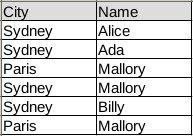In code the same table is:

You can then summarize the data using the groupby method. In our example there are two columns: Name and City.

The function .groupby() takes a column as parameter, the column you want to group on.
Then define the column(s) on which you want to do the aggregation.

This will count the frequency of each city and return a new data frame: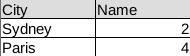The total code being:

## Example 2

The groupby() operation can be applied to any pandas data frame.
Lets do some quick examples.

The data frame below defines a list of animals and their speed measurements.

You can group by animal and the average speed.

If you have multiple columns in your table like so:

Then you can add the column like this:

## Example 3: Real World Data

The Iris flower data set contains data on several flower species and their measurements. You can load it the whole data set from a csv file like this:

You can read any csv file with the .read_csv() function like this, directly from the web.

To give you some insight into the dataset data:

You can retrieve the same with:

You can easily retrieve the minimum and maximum of a column

You’ve seen the basic groupby before. You can apply groupby while finding the average sepal width.

This then returns the average sepal width for each species.

If you want the minimum value for each sepal width and species, you’d use:

## Conclusion

We’ve covered the groupby() function extensively. You can now apply the function to any data frame, regardless of wheter its a toy dataset or a real world dataset.

You’ve learned:

• how to load a real world data set in Pandas (from the web)
• how to apply the groupby function to that real world data.

If you are interested in learning more about Pandas, check out this course:
Data Analysis with Python and Pandas: Go from zero to hero

You can use pandas to read data from an Excel file into a DataFrame, and then work with the data just like you would any other dataset.

To read an Excel file into a DataFrame using pandas, you can use the read_excel() function. The read_excel() function returns a DataFrame by default, so you can access the data in your DataFrame using standard indexing and slicing operations.

Pandas, a data analysis library, has native support for loading excel data (xls and xlsx). The method read_excel loads xls data into a Pandas dataframe:

If you have a large excel file you may want to specify the sheet:

Related course
Data Analysis with Python Pandas

The code below reads excel data into a Python dataset (the dataset can be saved below).

The dataframe can be used, as shown in the example below:

Dataset
For purpose of demonstration, you can use the dataset from: depaul.edu.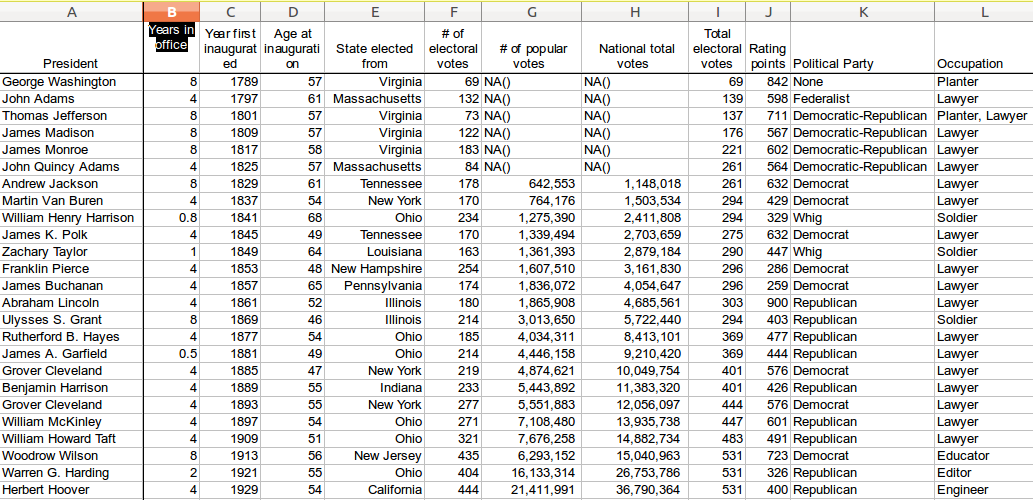A large dataset stored in XLS format

## visualize data with python

Get the xls data for this tutorial from: depaul.edu. This dataset contains a list of US presidents, associated parties,profession and more.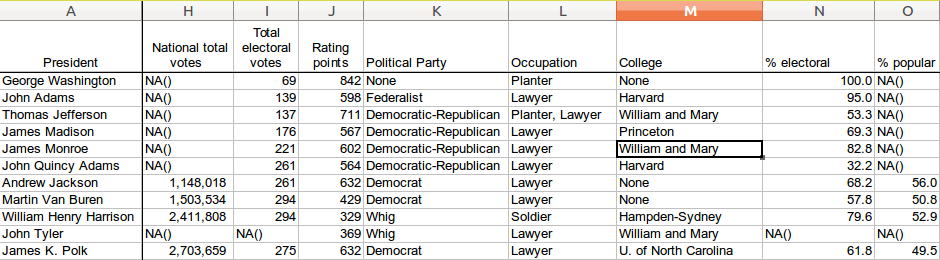Python Pandas Dataset

Related course
Data Analysis with Python Pandas

Beautiful Plots with Pandas
We can plot data of this large excel file with a few lines of code. We select the column “Occupation” for this demonstration using:

Full code: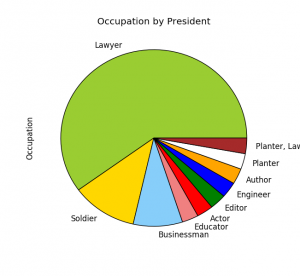Data cleaning and plotting
We can also plot the popularity by president. Before we do that, we need to clean some data: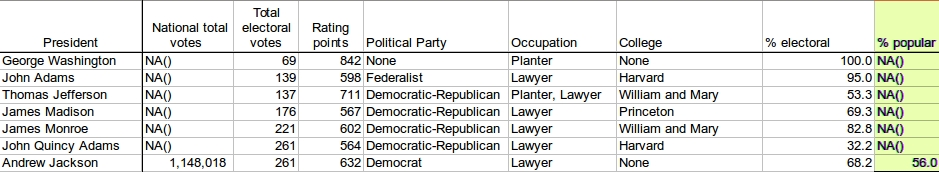Data cleaning with Pandas

Some of the cells do not contain numerical values, we can either remove them or replace them:

To plot the popularity: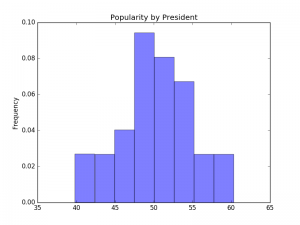Popularity by President

Pandas is a data analaysis module. It provides you with high-performance, easy-to-use data structures and data analysis tools.

Related course
Data Analysis with Python Pandas

We create a comma seperated value (csv) file:

Imported in excel that will look like this: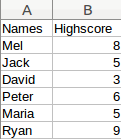Python Pandas example dataset

The data can be read using:

The first lines import the Pandas module. The read_csv method loads the data in a a Pandas dataframe that we named df.

Dataframes
A dataframe can be manipulated using methods, the minimum and maximum can easily be extracted: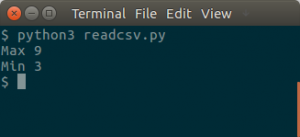Pandas on a dataset

The dataset in this example is very small, but a dataset can easily contain thousands or millions of records.